# Test: Waveguides - Electronics and Communication Engineering (ECE)

Test Description

## 20 Questions MCQ Test GATE ECE (Electronics) 2023 Mock Test Series - Test: Waveguides

Test: Waveguides for Electronics and Communication Engineering (ECE) 2023 is part of GATE ECE (Electronics) 2023 Mock Test Series preparation. The Test: Waveguides questions and answers have been prepared according to the Electronics and Communication Engineering (ECE) exam syllabus.The Test: Waveguides MCQs are made for Electronics and Communication Engineering (ECE) 2023 Exam. Find important definitions, questions, notes, meanings, examples, exercises, MCQs and online tests for Test: Waveguides below.
Solutions of Test: Waveguides questions in English are available as part of our GATE ECE (Electronics) 2023 Mock Test Series for Electronics and Communication Engineering (ECE) & Test: Waveguides solutions in Hindi for GATE ECE (Electronics) 2023 Mock Test Series course. Download more important topics, notes, lectures and mock test series for Electronics and Communication Engineering (ECE) Exam by signing up for free. Attempt Test: Waveguides | 20 questions in 60 minutes | Mock test for Electronics and Communication Engineering (ECE) preparation | Free important questions MCQ to study GATE ECE (Electronics) 2023 Mock Test Series for Electronics and Communication Engineering (ECE) Exam | Download free PDF with solutions
 1 Crore+ students have signed up on EduRev. Have you?
Test: Waveguides - Question 1

### Consider a 150 m long air-filled hollow rectangular wave guide with cutoff frequency 6.5 GHz. If a shortpulse of 7.2 GHz is introduced into the input end of theguide, the time taken by the pulse to return the inputend is

Detailed Solution for Test: Waveguides - Question 1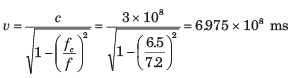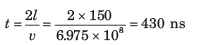Test: Waveguides - Question 2

### In an air-filled rectangular waveguide the cutoff frequencies for TM11 and TE03 modes are both equal to 12 GHz. Que: The dominant mode is

Detailed Solution for Test: Waveguides - Question 2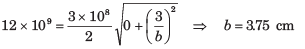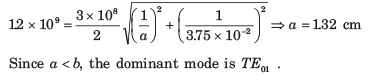Test: Waveguides - Question 3

### In an air-filled rectangular waveguide the cutoff frequencies for TM11 and TE03 modes are both equal to 12 GHz. Que:At dominant mode the cutoff frequency is

Detailed Solution for Test: Waveguides - Question 3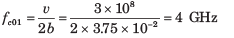Test: Waveguides - Question 4

For an air-filled rectangular waveguide given that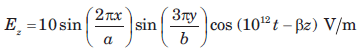If the waveguide has cross-sectional dimension a = 6 cm and b = 3 cm, then the intrinsic impedance of this mode is

Detailed Solution for Test: Waveguides - Question 4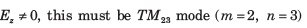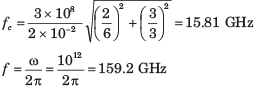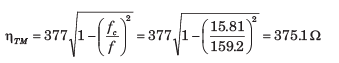Test: Waveguides - Question 5

In an air-filled waveguide, a TE mode operating at 6 GHz has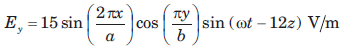Que: The cutoff frequency is

Detailed Solution for Test: Waveguides - Question 5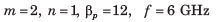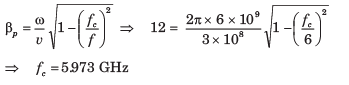Test: Waveguides - Question 6

In an air-filled waveguide, a TE mode operating at 6 GHz hasQue: The intrinsic impedance is

Detailed Solution for Test: Waveguides - Question 6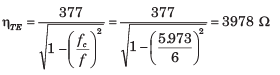Test: Waveguides - Question 7

Consider an air-filled rectangular wave guide with a = 2.286 cm and b = 1.016 cm. The y-component of the TE mode is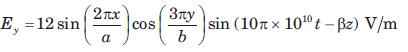Que: The propagation constant γ is

Detailed Solution for Test: Waveguides - Question 7

m = 2, n = 3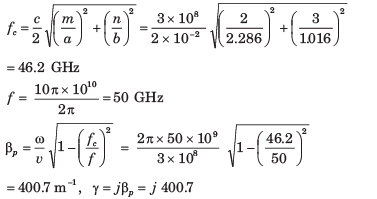Test: Waveguides - Question 8

Consider an air-filled rectangular wave guide with a = 2.286 cm and b = 1.016 cm. The y-component of the TE mode isQue: The intrinsic impedance is

Detailed Solution for Test: Waveguides - Question 8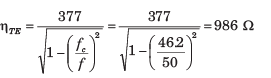Test: Waveguides - Question 9

Consider a air-filled waveguide operating in the TE12 mode at a frequency 20% higher than the cutoff frequency.

Que: The phase velocity is

Detailed Solution for Test: Waveguides - Question 9

v = c, f = 1.2 fc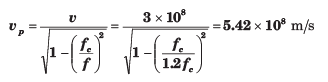Test: Waveguides - Question 10

Consider a air-filled waveguide operating in the TE12 mode at a frequency 20% higher than the cutoff frequency.

Que: The group velocity is

Detailed Solution for Test: Waveguides - Question 10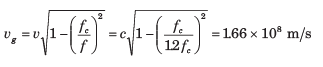Test: Waveguides - Question 11

A rectangular waveguide is filled with a polyethylene ( εr = 2.25 ) and operates at 24 GHz. The cutoff frequency of a certain mode is 16 GHz. The intrinsic impedance of this mode is

Detailed Solution for Test: Waveguides - Question 11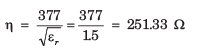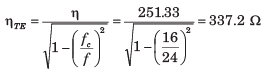Test: Waveguides - Question 12

own in fig. It has dielectric discontinuity as shown in fig. If the guide operate at 8 GHz in the dominant mode, the standing wave ratio is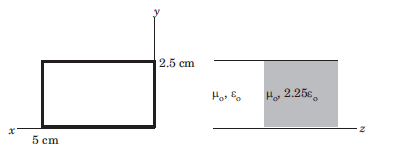Detailed Solution for Test: Waveguides - Question 12

Since a > b, the dominant mode is TE10 .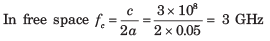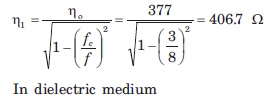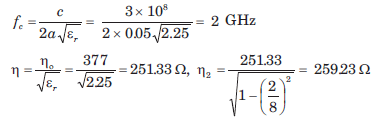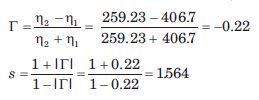Test: Waveguides - Question 13

Consider the rectangular cavity as shown in fig.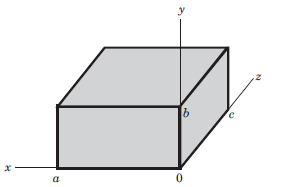Que: If a < b < c, the dominant mode is

Detailed Solution for Test: Waveguides - Question 13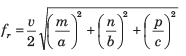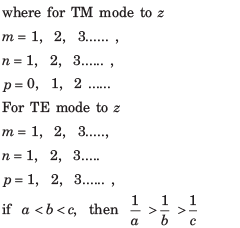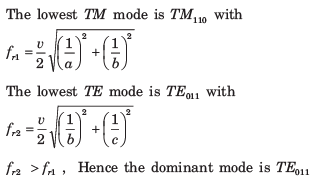Test: Waveguides - Question 14

Consider the rectangular cavity as shown in fig.Que: If a > b >  c, then the dominant mode is

Detailed Solution for Test: Waveguides - Question 14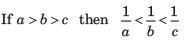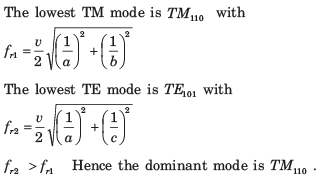Test: Waveguides - Question 15

Consider the rectangular cavity as shown in fig.Que: If a = c > b, then the dominant mode is

Detailed Solution for Test: Waveguides - Question 15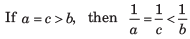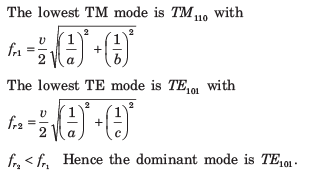Test: Waveguides - Question 16

The air filled cavity resonator has dimension a = 3 cm, b = 2 cm, c = 4 cm. The resonant frequency for the TM110 mode is

Detailed Solution for Test: Waveguides - Question 16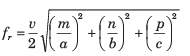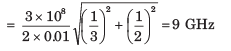Test: Waveguides - Question 17

A 6 cm x 4 cm rectangular wave guide is filled with dielectric of refractive index 1.25

Que: The range of frequencies over which single mode operation will occur is

Detailed Solution for Test: Waveguides - Question 17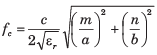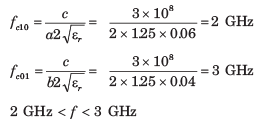Test: Waveguides - Question 18

A 6 cm x 4 cm rectangular wave guide is filled with dielectric of refractive index 1.25

Que: The range of frequencies, over which guide support both TE10 and TE01 modes and no other, is

Detailed Solution for Test: Waveguides - Question 18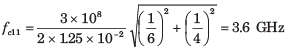3 GHz < f < 3.6 GHz

Test: Waveguides - Question 19

Two identical rectangular waveguide are joined end to end where a = 2b. One guide is air filled and other is filled with a lossless dielectric of εr. it is found that up to a certain frequency single mode operation can be simultaneously ensured in both guide. For this frequency range, the maximum allowable value of εr is

Detailed Solution for Test: Waveguides - Question 19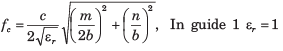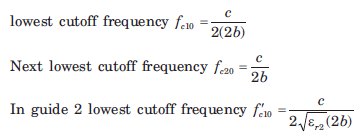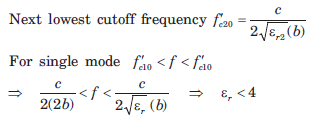Test: Waveguides - Question 20

A parallel-plate guide operates in the TEM mode only over the frequency range 0 < f < 3 GHz. The dielectric between the plates is teflon (εr = 2.1) .The maximum allowable plate separation b is

Detailed Solution for Test: Waveguides - Question 20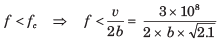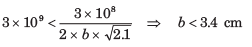## GATE ECE (Electronics) 2023 Mock Test Series

21 docs|263 tests(Scan QR code)